# Calculating Angular Momentum: Definition, Formula & Examples

An error occurred trying to load this video.

Try refreshing the page, or contact customer support.

Coming up next: Angular Momentum Practice Problems

### You're on a roll. Keep up the good work!

Replay
Your next lesson will play in 10 seconds
• 0:01 Angular Momentum Defined
• 0:42 Formula
• 0:56 Moment of Inertia
• 2:08 Angular Speed
• 2:31 Examples
• 5:56 Lesson Summary

Want to watch this again later?

Timeline
Autoplay
Autoplay
Speed

#### Recommended Lessons and Courses for You

Lesson Transcript
Instructor: Sharon Linde
Angular momentum is sometimes a tough concept to grasp, but with the right understanding and formula, it can be a snap. Let's explore its key concepts and give you some examples to help you understand.

## Angular Momentum Defined

Angular momentum is the rotational equivalent to linear momentum. Both concepts deal with how quickly something is moving and how difficult it is to change that speed. However, linear momentum had only two variables: mass and speed. Angular momentum starts out with a very similar equation, so it may initially look to be about the same complexity. As we'll see, it gets more complicated and involves more variables than linear momentum.

Angular momentum is the product of an object's moment of inertia and its angular velocity. Both quantities must be about the same axis, the rotation line.

## Formula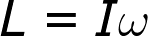In the above equation, L represents angular momentum. The moment of inertia is represented by I, and the angular speed is omega. Let's see how this equation works.

## Moment of Inertia

The term 'moment of inertia' may sound confusing, but I'll bet you already know the basic concepts involved in calculating it. Let's say you have two rods of wood. They both weigh the same amount and have the same diameter, but one of them is 1 foot long and the other is 10 feet long. Which one will you be able to make spin faster if you throw it overhand towards a target? Does it take more energy to spin a ball around your head if it is on a 2 foot rope or a 5 foot rope? What if you double the mass of the ball?

You knew the smaller rod and shorter rope were going to be easier to spin, didn't you? So you're already on your way to calculating the moment of inertia for an object. You may be used to the word 'moment' referring to time, but in math and physics, moment refers to torque or twisting. So the moment of inertia refers to how difficult an object is to twist around a particular axis. The moment of inertia of any object is determined by three factors: shape, mass and the axis of rotation.

Here's a chart explaining the moment of inertia for different shapes:

Shape Axis of Rotation Moment of Inertia
sphere center of sphere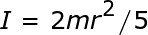point mass distance r from point mass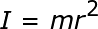solid cylinder axis of symmetry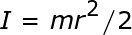rod (length L) center (not axis of symmetry)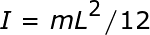rod (length L) one end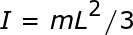## Angular Speed

The angular speed of an object is how quickly it is rotating about the axis of rotation. You can calculate the angular speed of an object if you know the linear speed of any point on it by using the equation omega = v / r. Omega is the angular speed, v is the linear velocity, and r is the distance from the axis of rotation.

## Examples

Let's look at a few examples to help us understand how to calculate angular momentum.

Example 1 - Point Mass

Ellen is spinning in a circle while attached to a rope with a 3 kg ball at the other end. The ball is traveling with a linear velocity equal to 10 m/s. Calculate the angular momentum of this ball.

The first thing to do here, as in many angular momentum problems, is to calculate the moment of inertia. This is an example of a point mass; in this situation, it doesn't matter what the shape of the weight is - only its mass is important. The calculations would be exactly the same if it were a cube, rectangular block or any irregular shape attached by the rope to Ellen.

I = (3 kg)(2 m)(2 m) = 12 kgm^2.

Now the angular speed:

Omega = v / r = (10 m/s) / (2 m) = 5s

Now with both values, we can determine the angular momentum.

L = Iw = (12 kgm^2)(5s) = 60 kgm^2/s

To unlock this lesson you must be a Study.com Member.

### Register to view this lesson

Are you a student or a teacher?

### Unlock Your Education

#### See for yourself why 30 million people use Study.com

##### Become a Study.com member and start learning now.
Back
What teachers are saying about Study.com

### Earning College Credit

Did you know… We have over 160 college courses that prepare you to earn credit by exam that is accepted by over 1,500 colleges and universities. You can test out of the first two years of college and save thousands off your degree. Anyone can earn credit-by-exam regardless of age or education level.